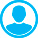PRIME NUMBER LAW. DEPENDENCE OF PRIME NUMBERS ON THEIR ORDINAL NUMBERS AND GOLDBACH – EULER BINARY PROBLEM USING COMPUTERAbstract (English):
The article considers the methods of defining and finding the distribution of composite numbers CN, prime numbers PN, twins of prime numbers Tw and twins of composite numbers TwCN that do not have divisors 2 and 3 in the set of natural numbers - ℕ based on a set of numbers like Θ = {6∙κ ± 1, κ ∈ ℕ}, which is a semigroup in relation to multiplication. There has been proposed a method of obtaining primes by using their ordinal numbers in the set of primes and vice versa, as well as a new algorithm for searching and distributing primes based on a closedness of the elements of the set Θ. It has been shown that a composite number can be presented in the form of products (6x ± 1) (6y ± 1), where x, y ℕ - are positive integer solutions of one of the 4 Diophantine equations: . It has been proved that if there is a parameter λ of prime twins, then none of Diophantine equations P (x, y, λ) = 0 has positive integer solutions. There has been found the new distribution law of prime numbers π(x) in the segment [1 ÷ N]. Any even number is comparable to one of the numbers i.e. . According to the above remainders m, even numbers are divided into 3 types, each type having its own way of representing sums of 2 elements of the set Θ. For any even number in a segment [1 ÷ ν], where ν = (ζ−m) / 6, , there is a parameter of an even number; it is proved that there is always a pair of numbers that are elements of the united sets of parameters of prime twins and parameters of transition numbers , i.e. numbers of the form with the same λ, if the form is a prime number, then the form is a composite number, and vice versa.

Keywords:
prime and composite numbers, parameters of primes, Diophantine equations, binary (strong) Goldbach – Euler, algorithm for solving the binary Goldbach – Euler problem.